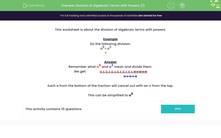# Divide Algebraic Terms with Powers

In this worksheet, students will divide algebraic terms with indices or powers.Key stage:  KS 3

Curriculum topic:   Algebra

Curriculum subtopic:   Use and Interpret Algebraic Notation

Difficulty level:#### Worksheet Overview

This activity is about the division of algebraic terms with powers.

Example

Do the following division.

a9÷ a3 =

Remember what a9 and a3 mean and divide them. We get:

a x a x a x a x a x a x a x a x a

a x a x a

Each a from the bottom of the fraction will cancel out with an a from the top leaving a6

This process can be simplified into the rule

a÷ an = am-n

Let's have a go at applying that rule in the questions that follow.

### What is EdPlace?

We're your National Curriculum aligned online education content provider helping each child succeed in English, maths and science from year 1 to GCSE. With an EdPlace account you’ll be able to track and measure progress, helping each child achieve their best. We build confidence and attainment by personalising each child’s learning at a level that suits them.

Get started